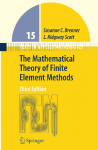# Finite Element Course

Lecturer: David Ham

In Autumn and Spring 2013-2014 I gave a series of lectures on the finite element method to PhD students from the Departments of Computing and Earth Science and Engineering. Each lecture is around 90 minutes.

## Lecture videos

The lectures are videoed and are publicly available. Each lecture has a link to the Imperial Panopto site and YouTube. You mileage may vary as to which offers better quality. There is a YouTube playlist and atom feed, as well as a Panopto atom feed.## Text and materials

For the first few lectures I follow Brenner and Scott The Mathematical Theory of Finite Element Methods quite closely. Imperial College has fortunately paid for PDF access to this book, so members of Imperial can obtain it from Springer Link.

## Lectures

### Lecture -1: Function spaces

This is a background lecture in which I cover the concept of vector spaces, and the idea that we can consider functions as vectors. The notes I used in this lecture are available here.

### Lecture 0: Finite element in 1D

In this lecture we cover sections 0.1-0.3 of Brenner and Scott. We discuss the weak form of a second order linear PDE and derive an abstract finite element problem. From this, we establish the existence and uniqueness of the finite element solution, and derive basic error constraints.

### Lecture 1: Implementing finite element in 1D

In this lecture we introduce the piecewise linear continuous function space, and show that it has the required approximation properties from the previous lecture. We then go through the process of assembling forms into matrices and vectors.

### Lecture 2: Sobolev spaces and weak derivatives

This week we covered several sections from chapter 1 of Brenner and Scott. In particular we defined weak derivatives and showed that we could use these to develop derivatives in function spaces important for the Finite Element method. During the lecture I mentioned a series of lectures on distributions as a part of a Fourier transforms course at Stanford. For those who want a more detailed exposition of distributions and derivatives, I really recommend lectures 12-14. The lectures are online here.

### Lecture 3: Constructing finite elements

In this lecture we looked at the classical Ciarlet description of finite elements and covered the role of the nodes in determining the basis functions. The material is primarily from chapter 3 of Brenner and Scott.

### Lecture 4: Finite element in more dimensions.

In the first half of this lecture, we discuss the Gâteaux derivative and the divergence operator. We establish the divergence theorem and some of the rules for integration by parts for functions over a multidimensional vector space. In the second half of the lecture, we applied these to derive the finite element form of Poisson’s equuation over a multidimensional domain.

### Lecture 5: Quadrature, Jacobians, and local assembly.

In this lecture we review numerical quadrature, before going on to formulate the actual assembly of a finite element operator on an element. We cover the evaluation of the change of coordinates from the physical element to the reference element, and introduce the idea of a non-affine change of coordinates.

### Lecture 6: Nonlinear problems.

This week we look at nonlinear variational problems, using the Burgers Equation as a simple example. We apply the Gâteaux derivative to linearise the problem and employ Newton’s method as a solver.

### Lecture 7: Boundary conditions and vector-valued spaces.

In the first of this week’s lectures we look at the imposition of strong (Dirichlet) boundary conditions through the modification of the residual. In the second half of the lecture we examine representations for vector-valued quantities in the finite element method.